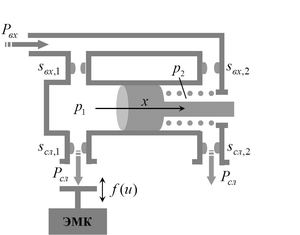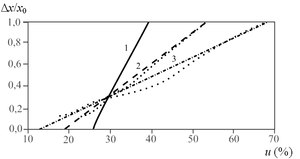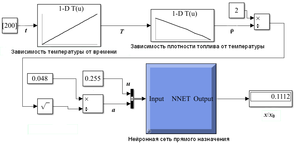ISSN 2658–5782
DOI 10.21662
Electronic Scientific Journalим. Р.Р. Мавлютова
УФИЦ РАНDenisova E.V., Chernikova M.A. The model of the servo piston of the fuel metering unit using matrix approach and neural network. Multiphase Systems. 13 (2018) 4. 107–111.
2018. Vol. 13. Issue 4, Pp. 107–111
URL: http://mfs.uimech.org/mfs2018.4.015,en
DOI: 10.21662/mfs2018.4.015
The model of the servo piston of the fuel metering unit using matrix approach and neural network
Denisova E.V., Chernikova M.A.
Mavlutov Institute of Mechanics, UFRC RAS, Ufa

### Abstract

In the paper, the model of a servo piston of a fuel metering unit based on a matrix approach using neural networks is considered. To develop the model of a servo piston, the dependence of the initial piston deviation on the control signal for different values of the nozzles is used. This dependence is represented in the form of a matrix and is used in the neural network. This approach allows describing the movement of the servo piston with a sufficient degree of accuracy. As a record of change squares adjustment of the nozzles is a source of parametric uncertainty in the operation of the automatic control system can lead to a drop in the quality of control, such accounting is relevant. The model of the servo piston is proposed to be used in the structure of the automatic control system for a gas turbine engine and for semi-natural stands.

Keywords

fuel metering unit,
servo piston,
matrix approach,
neural network,
simulation

## Article outline

Purpose: Development of the model of a servo piston of a fuel metering unit using a matrix approach and a neural network.

Methods: To develop the model of a servo piston of a fuel metering unit, two methods are used: the matrix approach and the neural network algorithm. The servo piston model was studied in the framework of the dynamic model of the fuel dosing unit, the solution of which is determined by a system of nonlinear differential equations. Since there are structural features of the fuel metering unit, a separation of static characteristics was obtained, which is unacceptable when the automatic control system of an unmanned aerial vehicle is operating. Therefore, an information parameter was introduced characterizing the degree of possible separation of the static characteristics of the servo piston. Thus, it became possible to represent the deviation of the servo piston from the initial position in the form of a matrix for subsequent implementation using a neural network.

Results: An information parameter was introduced that allows the deviation of the servo piston from the initial position to be represented using the matrix approach, taking into account the degree of possible parametric uncertainty. To reduce the computation time and build faster models of a servo piston of a fuel metering unit, a neural network was synthesized using a matrix approach in data processing. Using the obtained neural network, in the Simulink simulation environment, a model of a servo piston is implemented. The results of the work of the obtained model are shown, which demonstrated the adequacy of the model operation in comparison with experimental data.

Findings: The resulting model can be used to simulate the entire automatic control system for an unmanned aerial vehicle, which will improve the quality of control of the system as a whole.

## References

1. Насибуллаева Э.Ш., Денисова Е.В. Динамическая модель агрегата дозирования топлива в силовую установку беспилотного летательного аппарата // Вестник РФФИ. 2012. No 2–3(74–75). С. 60–68. (http://www.rfbr.ru/rffi/ru/bulletin/o_1774291#62)
2. Денисова Е.В., Насибуллаева Э.Ш., Насибуллаев И.Ш. Исследование динамических процессов в элементах топливной автоматики // Мехатроника, автоматизация, управление. 2014. No 5(158). С. 31–36. (http://novtex.ru/mech/mech2014/annot05.html#5)
3. Денисова Е.В., Мигранов А.Б., Черникова М.А. Матричный подход к моделированию газотурбинного двигателя с использованием нейронных сетей // Современные наукоемкие технологии. 2018. No 3. С. 35–40. (https://www.top-technologies.ru/ru/article/view?id=36933)
4. Методы робастного, нейро-нечеткого и адаптивного управления / Под редакцией Н.Д. Егупова. МГТУ им. Н.Э. Баумана, 2001. 744 с.
5. Хайкин С. Нейронные сети: полный курс, 2-е издание. Пер. с англ. М.: Издательский дом «Вильямс», 2006. 1104 с.